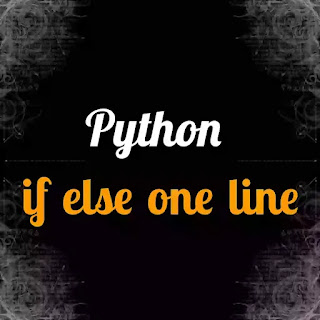# Python if else one line - codebugfree

In this section we learn if else one line python code how to write. It is quit difficult to write this if else one line python program. You know the basic of comprehension. make the code one liner.

Comprehension is different types they are List comprehension, Dictionary comprehension. One liner code is not readable for beginner python programmer. Create a lot of confuse in one liner code. Advice for beginner doesn't use comprehension to write if else in one line. When you reach in intermediate level than you try to write one liner codePython if else one line

Beginner always ask question. Why advance python programmer write the program if else in one line. They know one liner program is not readable.

Because Advance programmer has not focus in code readability programmer focus the code length and most important code running time. 1 mili second time difference is also effect the hosting and the computer Ram data so, it is important things to focus Advance programmer so they choose the comprehension to reduce the length of code.

### How to write if else one line in python

First we see the multiline if else. Then one line if else see with example and Code explination.

Example:

``````user_input = int(input("Enter a number: "))
if user_input%2==0:
print("this is even number")
else:
print("this is odd")``````

First we input from user and a number type casting in int. Type casting is the change one datatype keyword to another  keyword like sting to int, int to boolen, int to decimal, strint to float. use if statement to checking the user input number reminder is 0 or not if 0 than print it is even. else than print it is odd.

INOUTPUT

``````Enter a number: 7
this is odd``````

Now see the example of if else in one line it is to difficult to read.

Example if else in one line:

``````user_input = int(input("Enter a nummber: "))
[user_input if user_input%2==0  else print("it is not odd")]``````

We recieve the input from user in number that number is int. if user input number reminder is 0 than stop the program. if reminder is 1 than print the else.

INOUTPUT:

``````Enter a nummber: 41
it is  odd``````

One practice problem for intermediate python programmer. This is question Get a user age or date of birth . if input user age that your program print date of birth or input date of birth print user age. Note don't use any module like date and time.

``````today_date = 2021
user_age = (input("Enter your age or date_0f_birth: "))
print(user_age)
if len(user_age)==2:
age_calcu = 2021 - int(user_age)
print(f"your date of birth is {age_calcu}")
find_100 = 100 + age_calcu
print(f"you make century birthday in {find_100}")

elif len(user_age)==1:
print("you is to small to use our program")
age_calcu = 2021 - int(user_age)
print(f"your date of birth is {age_calcu}")
find_100 = 100 + age_calcu
print(f"you make century birthday in {find_100}")
elif len(user_age)==4:
print("You enter your date of birth")
if today_date greater sing int(user_age):
print ("you not born")
else:
date_calcu = today_date - int(user_age)
find_100 = int(user_age) + 100
print(f"you make century birthday in {find_100}")
else:
print("you input a invalid word")``````

First set the date of year. user input his date of birthday or age. checking the length of user input 2 for age and 4 for date of birth day . If 2 then user input his age subtraction between date of this year and age answer is user date of birth.

User input date of birth then subtraction  between this year date and use date of birth. To calculate the user age. Also calculate in which year user hit 100 year old

INOUTPUT with age

``````Enter your age or date_0f_birth: 18
18
your date of birth is 2003
you make century birthday in 2103``````

IOUTPUT with date of birth

``````Enter your age or date_0f_birth: 2003
2003
You enter your date of birth